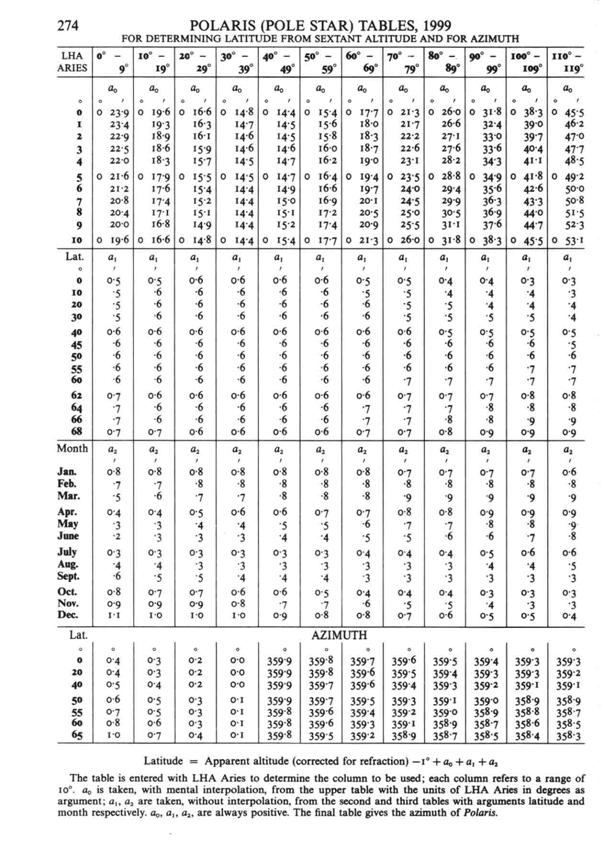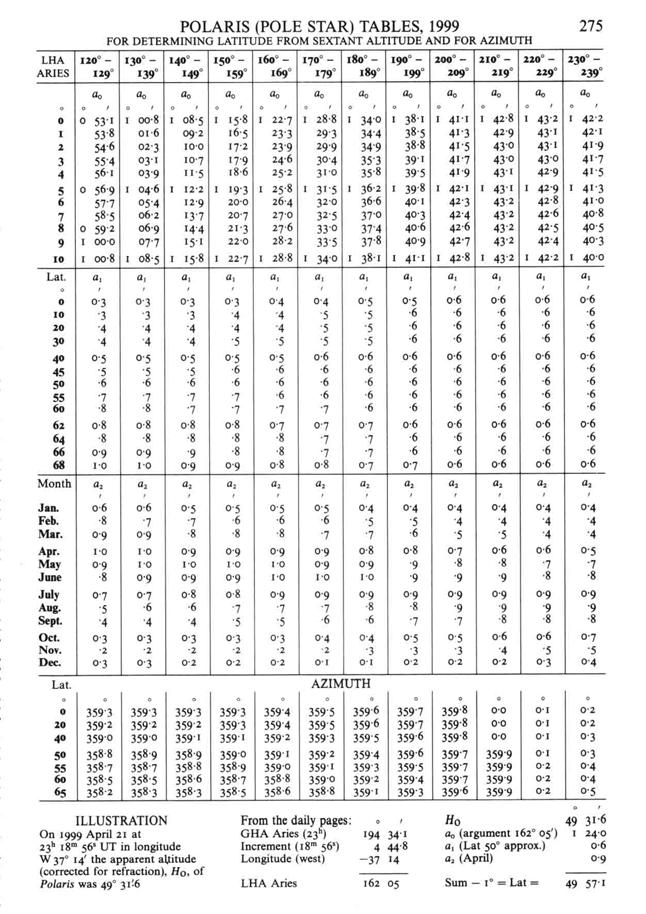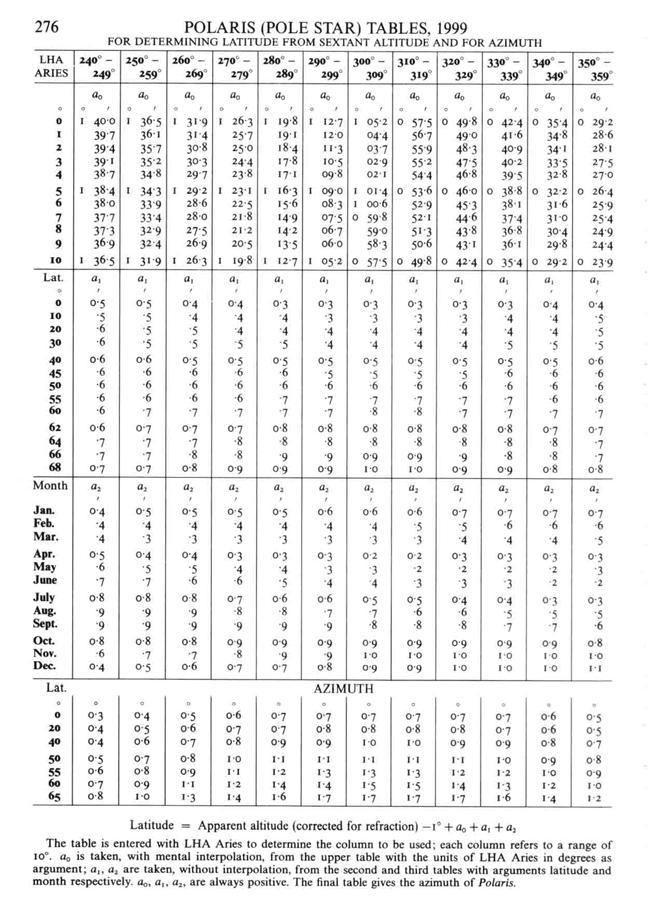Back to Nautical Solar Sys. Hour Angles PZX Triangles Dip Amplitude Day Ex Meridian Exercises Pole Star Naut. Almanac

Pole Star

Latitude by Altitude

The Pole Star so named because it points due north, is actually away from the astronomical pole by about 57’ and describes a small circle (clockwise) around the Pole every day, the azimuth changes from 002˚ to 358˚. During the day (24h) the pole star bears north only on two occasions when it crosses the meridian above and below the pole.

The Pole Star is on the meridian at Upper Transit its Local Hour Angle is 0˚. At the lower transit it is 180˚, when it is at right angles to the meridian due WEST of the pole it is 90˚ and when it is at right angles to the meridian due EAST of the pole it is 270˚.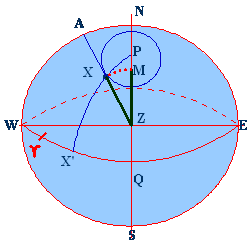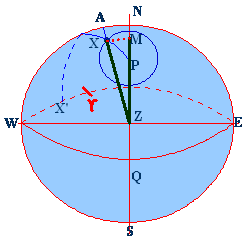In the figures above we see the LHA of the Pole Star (angle ZPX) as 050˚ and 130˚.

AX is the true altitude of the pole star and ZX is the zenith distance. NM is made equal to AX and is equal to the true altitude.

When this done we see that the correction to be applied to NM to get the Latitude would be MP.

This correction MP would be applied to the true altitude if the LHA were between 90˚ and 270˚, and it would be subtracted if the LHA were between 0˚ to 90˚ or between 270˚ to 360˚.

Using the Almanac for obtaining the corrections:

To obtain the observed Latitude is quite simple. The following steps are taken:

Correct the observed altitude to True altitude

Subtract 1˚ from this True Altitude

Add the three corrections: a0, a1, a2

The above converts the True altitude into the Latitude.

Entering the Tables:

LHA (Aries) found by:

LHA (Aries) = GHA (Aries) +/- Longitude [Longitude WEST (-) and Longitude EAST (+)]

First Correction a0: This is given in the Almanac for every degree of Local Hour Angle (Aries) and abreast of the degree line.

Second Correction a1:  This is given under the nearest ten degrees of LHA (Aries) and abreast the nearest Latitude. The altitude of the star gives the approx Latitude.

Third Correction a2: This is given under the nearest ten degrees of LHA (Aries) and abreast of the Month of observation.

a0 is computed in the Almanac using mean values for Latitude and the star’s SHA and Declination, then 58.8’ is added.

a1 is the adjustment for observer’s Latitude and then 0.6’ is added.

a2 is the adjustment for changes in declination and SHA and then 0.6’ is added

a0 + a1 + a2 = 1˚ so that,

Latitude = True Altitude – 1˚ + a0 + a1 + a2

EXTRACTS FROM THE NAUTICAL ALMANAC